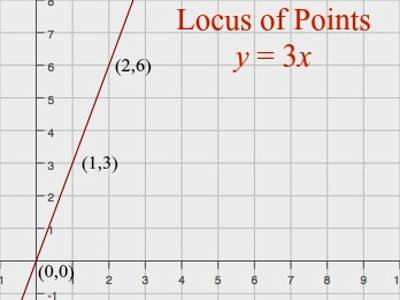The definitions of a plane curve like circle, parabola, ellipse etc. are based on the concept of the locus. For example, the locus of points that are 1cm from the origin is a circle of radius 1cm centered on the origin, since all points on this circle are 1cm from the origin.

If a point moves on a plane satisfying some given geometrical condition then the path traced out by the point in the plane is called its locus. By definition, a locus is determined if some geometrical condition is given. Evidently, the co-ordinate of all points on the locus will satisfy the given geometrical condition. The algebraic form of the given geometrical condition which is satisfied by the co-ordinate of all points on the locus is called the equation to the locus of the moving point. Thus, the coordinates of all points on the locus satisfy its equation of locus: but the coordinates of a point which does not lie on the locus, do not satisfy the equation of locus. Conversely, the points whose coordinates satisfy the equation of locus lie on the locus of the moving point.

Suppose X and Y are two fixed points in the two-dimensional coordinate plane. If a point M moves on this plane in such a manner that its distance from the points X and Y are always equal, then the point M will trace out a definite path on the plane.Examples of Locus of a Moving Point:

1. A point moving in such a manner that three times of distance from the x-axis is greater by 7 than 4 times of its distance from the y-axis; find the equation of its locus.

Solution:

Let P (x, y) be any position of the moving point on its locus. Then the distance of P from the x-axis is y and its distance from the y-axis is x.

By problem, 3y – 4x = 7,

Which is the required equation to the locus of the moving point.

1. Find the equation of the locus of a moving point which is always equidistant from the points (2, -1) and (3, 2). What curve does the locus represent?

Solution:

Let A (2, -1) and B (3, 2) be the given points and (x, y) be the

co-ordinates of a point P on the required locus. Then,

PA2 = (x – 2)2 + (y + 1)2 and PB2 = (x – 3)2 + (y – 2)2

By problem, PA = PB or, PA2 = PB2

or, (x – 2)2 + (y + 1)2 = (x – 3)2 + (y – 2)2

or, x2 – 4x + 4 + y2 + 2y + 1 = x2 – 6x + 9 + y2 – 4y + 4

or, 2x + 6y = 8

or, x + 3y = 4 ……… (1)

Which is the required equation to the locus of the moving point.

Clearly, equation (1) is a first-degree equation in x and y; hence, the locus of P is a straight line whose equation is x + 3y = 4.

Information Source: